Qian Dong(董乾)1, Xu-Ri Yao(姚旭日)2, †, Xin Liu(刘歆)3,4, Bing Liu(刘冰)2, and Guang-Jie Zhai(翟光杰)2,4$1 State Key Laboratory of Computer Science, Institute of Software, Chinese Academy of Sciences, Beijing 100190, China 2 Key Laboratory of Electronics and Information Technology for Space Systems, National Space Science Center, Chinese Academy of Sciences, Beijing 100190, China 3 State Key Laboratory of Scientific and Engineering Computing, Academy of Mathematics and Systems Science, Chinese Academy of Sciences, Beijing 100190, China 4 University of Chinese Academy of Sciences, Beijing 100049, China  Abstract A traditional single-pixel camera needs a large number of measurements to reconstruct the object with compressive sensing computation. Compared with the 1/0 matrices in classical measurement, the 1/−1 matrices in the complementary measurement has better property for reconstruction computation and returns better reconstruction results. However, each row of the 1/−1 matrices needs two measurements with the traditional single-pixel camera which results into double measurements compared with the 1/0 matrices. In this paper, we consider the pseudo complementary measurement which only takes the same amount of measurements with the row number of some properly designed 1/0 matrix to compute the total luminous flux of the objective and derives the measurement data of the corresponding 1/−1 matrix in a mathematical way. The numerical simulation and experimental result show that the pseudo complementary measurement is an efficient tool for the traditional single-pixel camera imaging under low measurement rate, which can combine the advantages of the classical and complementary measurements and significantly improve the peak signal-to-noise ratio. Keywords: computational imaging single-pixel camera Received: 25 May 2020 Revised: 08 July 2020 Accepted manuscript online: 25 August 2020 Fund: the National Key Research and Development Program of China (Grant No. 2018YFB0504302), the Youth Innovation Promotion Association of Chinese Academy of Sciences, and the National Natural Science Foundation of China (Grant Nos. 11701545, 11971466, and 11991021). Corresponding Authors: †Corresponding author. E-mail: yaoxuri@nssc.ac.cn #### Cite this article: Qian Dong(董乾), Xu-Ri Yao(姚旭日), Xin Liu(刘歆), Bing Liu(刘冰), and Guang-Jie Zhai(翟光杰)$ Pseudo complementary measurement for traditional single-pixel cameras 2020 Chin. Phys. B 29 114202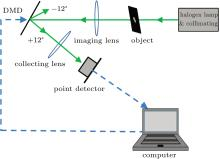Fig. 1.  Single-pixel camera.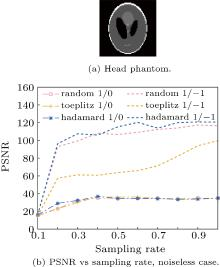Fig. 2.  The reconstruction quality versus sampling rate of different matrices for head phantom picture. In (b), the “random”, “toeplitz” and “hadamard” mean the random, Toeplitz and Hadamard matrices, respectively; the “1/0” and “1/−1” mean the 1/0 and 1/−1 matrices, respectively. The “hadamard−1/0” matrix is made with the 1 part of the corresponding Hadamard matrix. The sampling rates are taken 0.1, 0.2, 0.3, …, 1.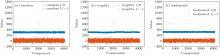Fig. 3.  The components in the 2048th row of AT A of different matrices, where A ∈ ℝ1228 × 4096.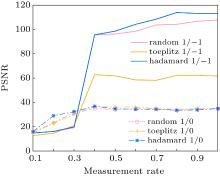Fig. 4.  Peak signal-to-noise ratio (PSNR) vs measurement rate of different measurements (matrices), noiseless case. The legends are the same as the ones in Fig. 2. The measurement rates are taken as 0.1, 0.2, 0.3, …, 1.Algorithm 1:  Matrix generation based on Assumptions 1 and 2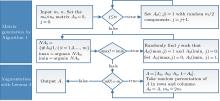Fig. 5.  The flow chart based on Algorithm 1 and Lemma 3.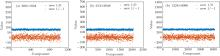Fig. 6.  The components in the 1st row of AT A of the matrices. The dimension of A is listed in the sub-figures. The “new−1/0” matrix is designed by Algorithm 1 and Lemma 3, and the “new−1/−1 matrix” is computed based on Eq. (4).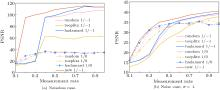Fig. 7.  PSNR vs measurement rate with the pseudo complementary measurement. The “new−1/−1” matrix is generated by Algorithm 1 and the other legends are the same as the ones in Fig. 4. The measurement rates are taken as 0.1, 0.2, 0.3, …, 1.Table 1.  The reconstructed PSNR of the head phantom in Fig. 1(a).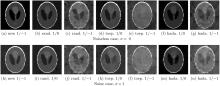Fig. 8.  The reconstructed image of the head phantom in Fig. 2(a). Here the measurement rate is 0.3, and σ is the standard deviation of the noise. The “new 1/−1” matrix is generated by Algorithm 1; the “rand.”, “toep.” and “hada.” mean the random, Toeplitz and Hadamard matrix respectively; the “1/0” and “1/−1” mean the 1/0 and 1/−1 matrices, respectively.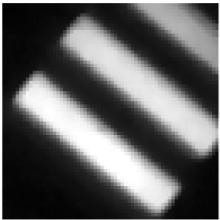Fig. 9.  The original image in the experiment.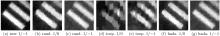Fig. 10.  The reconstructed image of Fig. 9. Here the measurement rate is 0.3. The “new 1/−1” matrix is generated by Algorithm 1; the “rand.”, “toep.” and “hada.” mean the random, Toeplitz and Hadamard matrix respectively; the “1/0” and “1/−1” mean the 1/0 and 1/−1 matrices, respectively.Table 2.  The reconstructed PSNR of Fig. 2(a).